﻿ 线索二叉树学习笔记

### 线索二叉树学习笔记

2021-11-23 0:57:46

# 线索二叉树的原理

记ptr指向二叉链表中的一个结点，以下是建立线索的规则：

（1）如果ptr->lchild为空，则存放指向中序遍历序列中该结点的前驱结点。这个结点称为ptr的中序前驱；

（2）如果ptr->rchild为空，则存放指向中序遍历序列中该结点的后继结点。这个结点称为ptr的中序后继；

（1）当k的左（或右）指针指向前驱（或后继）时，则置k的ltag（或rtag）为1，

（2）而当它们指向真正的子结点时，则k的ltag（或rtag）为0。

（3）只有当k是按中序的最前（后）结点时，k的左（右）指针才为空，同时k的ltag（rtag）取值为0。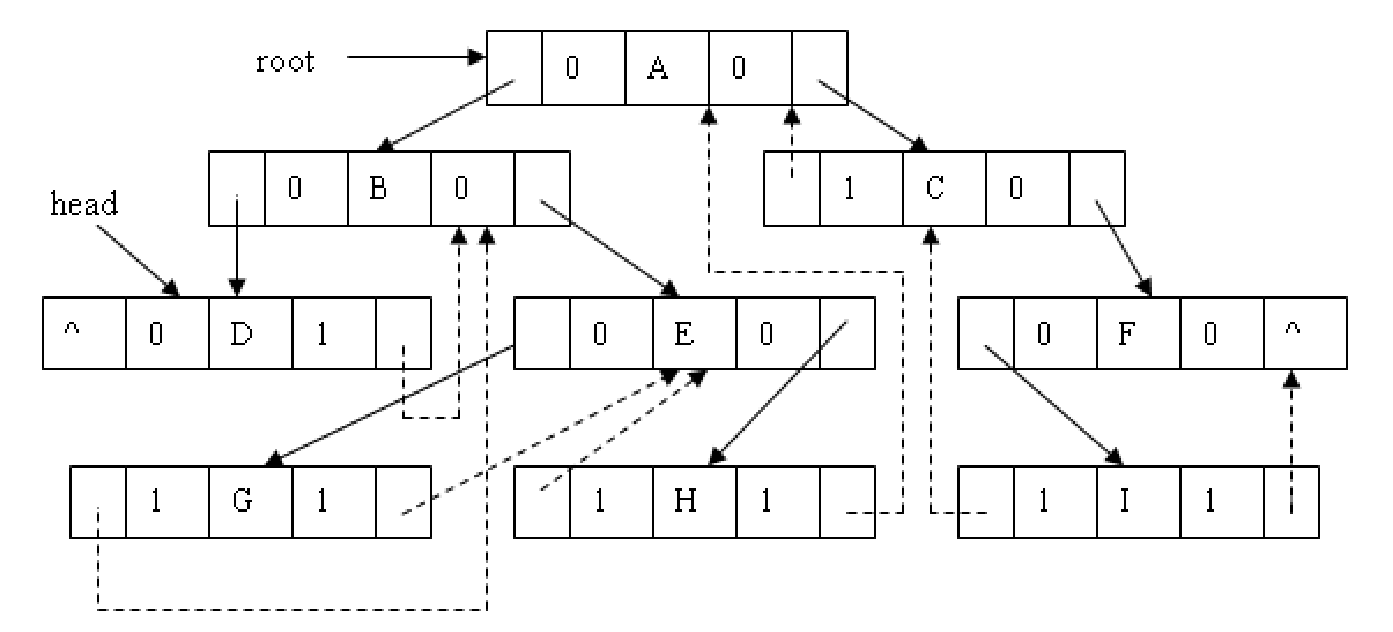# 二叉线索树存储结构定义

``````/* 二叉树的二叉线索存储结构定义*/
template <class T>
struct node {
T data;                                            //结点数据
node<T>* lchild, * rchild;                         //左右孩子指针
int ltag, rtag;                                    //左右标志
};
node<class T> BitNode,*BiTree;
``````

# 二叉线索树的实现

线索化的实质就是将二叉链表中的空指针改为指向前驱或后继的线索。由于前驱和后继信息只有在遍历该二叉树时才能得到，所以，线索化的过程就是在遍历的过程中修改空指针的过程。

``````//中序遍历进行中序线索化
{
if(p)//p！=NULL时执行
{
//===
if(!p->lchild)           //没有左孩子
{
p->ltag = 1;    //前驱线索
p->lchild = pre; //左孩子指针指向前驱
}
if(!pre->rchild)     //没有右孩子
{
pre->rtag = 1;  //后继线索
pre->rchild = p; //前驱右孩子指针指向后继(当前结点p)
}
pre = p;
//===
}
}``````

# 遍历二叉线索树

``````//t指向头结点，头结点左链lchild指向根结点，头结点右链rchild指向中序遍历的最后一个结点。
//中序遍历二叉线索树表示二叉树t
int InOrderThraverse_Thr(BiTree t)
{
BiTree p;
p = t->lchild;                               //p指向根结点
while(p != t)                               //空树或遍历结束时p == t
{
while(p->ltag == 0)                       //当ltag = 0时循环到中序序列的第一个结点
{
p = p->lchild;
}
printf("%c ", p->data);                      //显示结点数据，可以更改为其他对结点的操作
while(p->rtag == 1 && p->rchild != t)
{
p = p->rchild;
printf("%c ", p->data);
}

p = p->rchild;                         //p进入其右子树
}

return OK;
}``````

# 线索树上的插入操作

### 1、把q所指的结点插在p所指的结点按中序的前面

``````//把q所指的结点插在p所指的结点按中序的前面
template <class T>
void left_insert(node<T>* p, node<T>* q, node<T>** p_head) {//传进来的q只有data
node<T>* r;
if (p->ltag == 1 || p->lchild == NULL) {//p没有左孩子
q->lchild = p->lchild;
q->ltag = p->ltag;
q->rchild = p;       //p为q的后继
q->rtag = 1;
p->lchild = q;        //q作为p的左孩子
p->ltag = 0;
if (q->lchild == NULL) *p_head = q;
}
else {       //否则，q作为p的原来的前驱的右孩子
r = pred(p);//找到p的前驱
q->rchild = r->rchild;     q->rtag = r->rtag;
q->lchild = r;     q->ltag = 1;
r->rchild = q;     r->rtag = 0;
}
}

//在中序线索树中找结点t的前驱
template <class T>
node<T>* pred(node<T>* t) {
if (t->ltag == 1 || t->lchild == NULL) return t->lchild;
t = t->lchild;
while (t->rtag == 0) t = t->rchild;  //不会出现t->rchild == NULL
return t;
}``````

### 2、把q所指的结点插在p所指的结点按中序的后面

``````//把q所指的结点插在p所指的结点按中序的后面
template <class T>
void right_insert(node<T>* p, node<T>* q) {//p没有右孩子则q作为p的右孩子
node<T>* r;
if (p->rtag == 1 || p->rchild == NULL)
{
q->rchild = p->rchild;     q->rtag = p->rtag;
q->lchild = p;             q->ltag = 1;
p->rchild = q;             p->rtag = 0;
}
else {     //否则q作为p的后继的左孩子
r = succ(p);    //找到p的后驱
q->lchild = r->lchild;     q->ltag = r->ltag;
q->rchild = r;    q->rtag = 1;    r->lchild = q;     r->ltag = 0;
}
}
``````

# 线索树上的删除操作

### 以删除t的右子女r为例，分4种情况：

（1）r是叶子结点：r的后继s如果存在则s左孩子非空，不需调整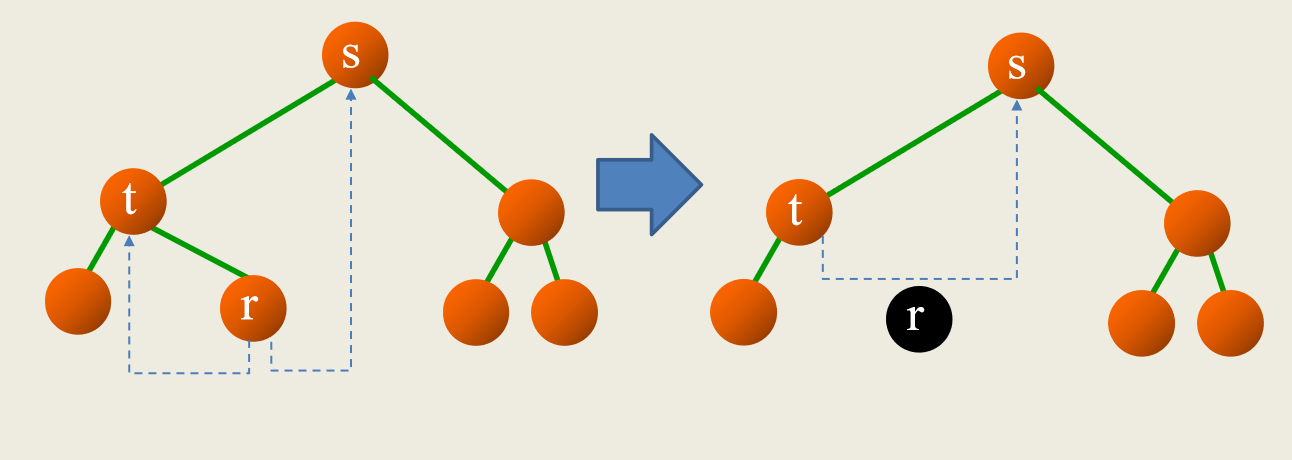（2）r的左孩子空：要让r的右子树中序第一个结点p（原来的前驱为r）记住r的前驱（实际上就是t）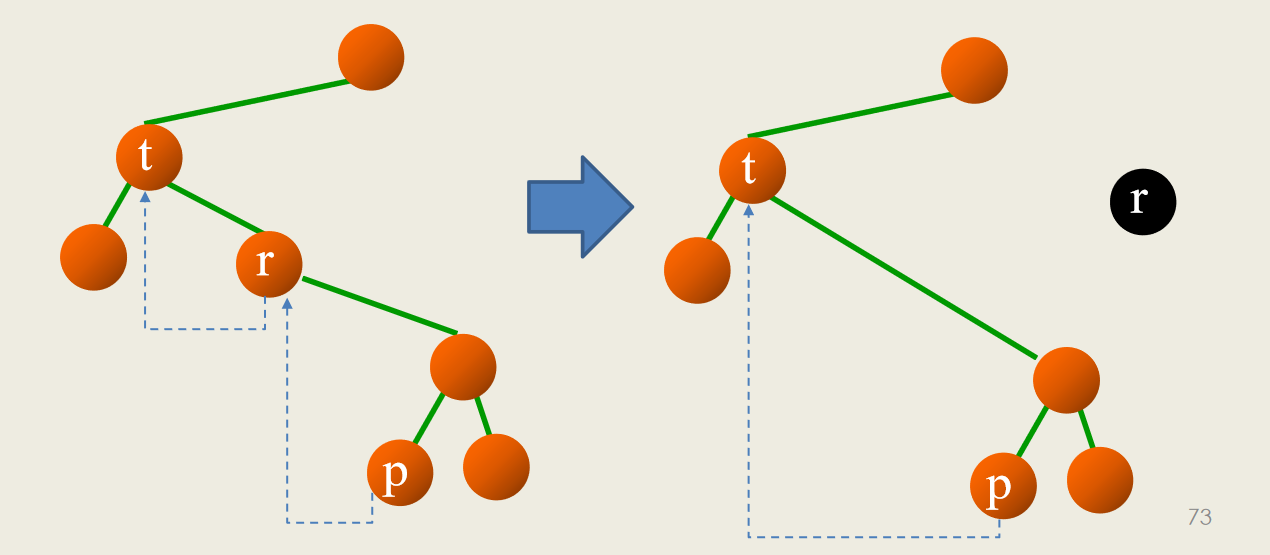（3）r的右孩子空：要让r的左子树中序最后结点p记住r的后继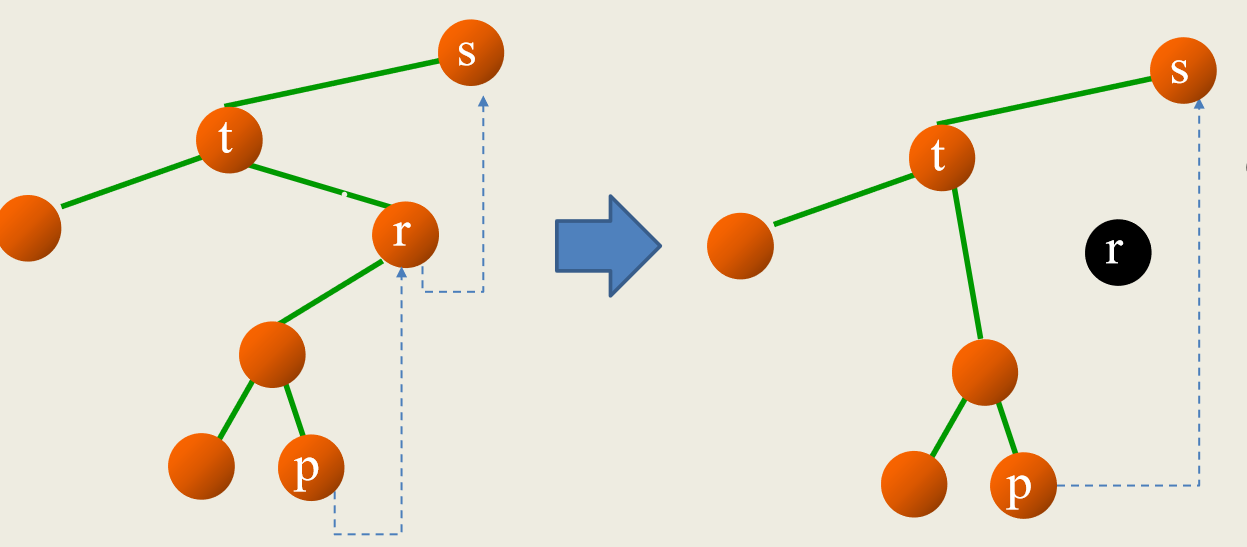（4）r同时有左右子树：把r的右子树接到r的左子树中序最后结点p之后（作为p的右子树），让r的右子树中序第一个结点q的左孩子指回p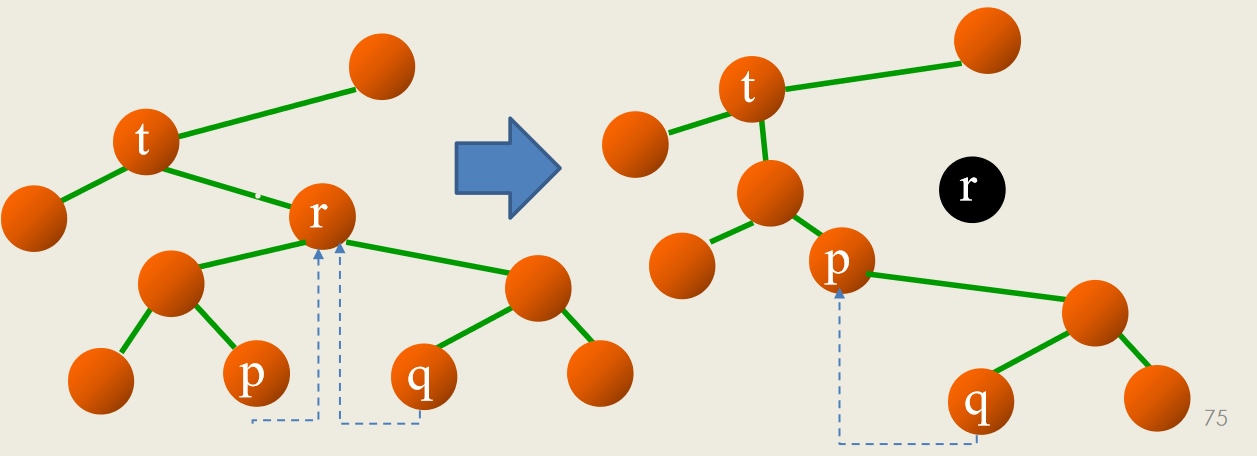（具体代码实现后续再补上。。。）